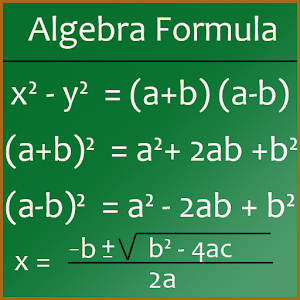# Maths Algebra Formula - Lumos Educational App Store3.91
Price -
\$

#### DESCRIPTION:

The app lists all the important Maths Algebra formulas. Its very useful for student to save valuable time. This App contains following formulas : 1) Arithmetic Progressoin 2) Arithmetic Operatopms 3) Law of Exponent 4) Factoring Formula 5) Absolute Value 6) Logarithms Properties 7) Complex Numbers 8) Radical Properties 9) Inequalities Properties 10) Quadratic Formula 11) Rules of Zeros

#### OVERVIEW:

Maths Algebra Formula is a free educational mobile app By simpleApps.It helps students in grades 8 practice the following standards .

This page not only allows students and teachers download Maths Algebra Formula but also find engaging Sample Questions, Videos, Pins, Worksheets, Books related to the following topics.

8

Developer: simpleApps

Software Version: 1.3

Category: Education

### RELATED APPS:EdSearch WebSearch